# Descartes oval

(diff) ← Older revision | Latest revision (diff) | Newer revision → (diff)

A plane curve for which the distances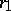and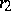between any pointof the curve and two fixed pointsand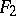(the foci) are related by the non-homogeneous linear equation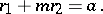A Descartes oval may be defined by means of the homogeneous linear equation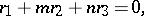where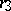is the distance to the third focus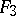located on the straight line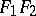. In the general case, a Descartes oval consists of two closed curves, one enclosing the other (see Fig.). The equation of a Descartes oval in Cartesian coordinates is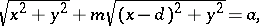whereis the length of the segment. Ifand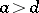, the Descartes oval is an ellipse; if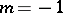and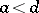, it is a hyperbola; if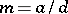, it is a Pascal limaçon. First studied by R. Descartes in the context of problems of optics .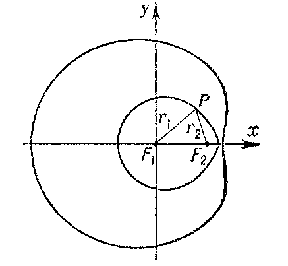Figure: d031340a

How to Cite This Entry:
Descartes oval. Encyclopedia of Mathematics. URL: http://encyclopediaofmath.org/index.php?title=Descartes_oval&oldid=16712
This article was adapted from an original article by E.V. Shikin (originator), which appeared in Encyclopedia of Mathematics - ISBN 1402006098. See original article